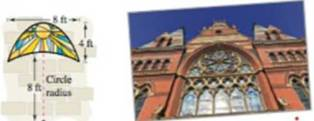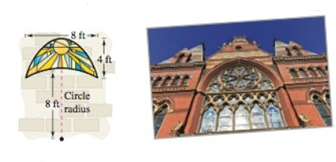Chapter 10.1, Problem 71E

Chapter
Section
Textbook Problem

# ArchitectureA church window it bounded above by a parabola and below by the arc of a circle (see figure) Find the area of the window.To determine

To calculate: Area of the window of the church bounded by the parabola.Explanation

Given: A church window is formed by a parabola bounded above and the arc of a circle below. The figure of the Church window is shown below.

Formula used:

Area of the window = 44 Area bounded between the parabola and the circle dx.

Calculation: The radius of the circle is provided as 8ft and if the circle is centred at the origin then the equation of the circle is, x2+y2=64. Now any point on the circle satisfy the equation of the circle. Since the gap between the two points on the parabola is 8ft. Their half becomes 4ft. That means, the points are 4ft each on either side of the origin. Let the point of intersection between the parabola and the circular arc is (4, h). Since the point satisfies the equation of parabola, so it can be substitute in the equation of circle to get the value of h. Therefore, 16+h2=64, that means h=48, that means, 4√3. Therefore, the tip of the parabola is at the point (0, 4+4√3), that is (0, 10.93). Therefore, the parabola has the equation of the type x2=4p(y10.93). Since the point (4, 4√3) lies on the parabola, so substitute the point on the curve, we have p = 1. Therefore, the equation of the parabola is given by x2=4(y10.93). Now the area bounded by the window is given by area between the curves for parabola and the circle, that means,

Area of the window = 44 Area bounded between the parabola and the circle dx

44(10

### Still sussing out bartleby?

Check out a sample textbook solution.

See a sample solution

#### The Solution to Your Study Problems

Bartleby provides explanations to thousands of textbook problems written by our experts, many with advanced degrees!

Get Started

#### Find more solutions based on key concepts